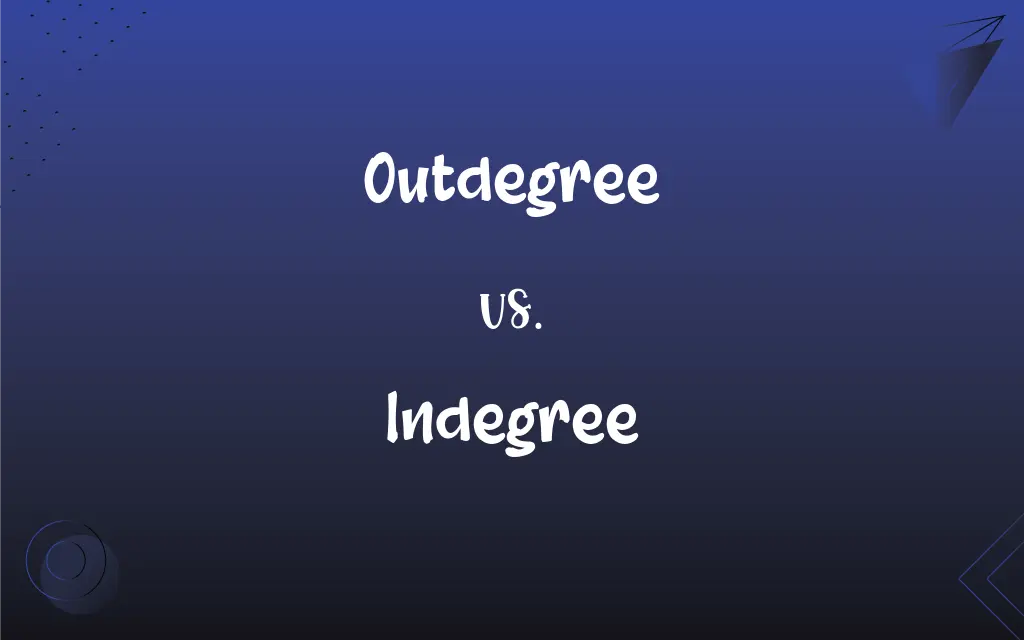# Outdegree vs. Indegree: What's the Difference?Edited by Huma Saeed || By Sawaira Riaz || Updated on September 29, 2023
Outdegree refers to the number of edges directed away from a vertex in a directed graph, while indegree refers to the number of edges directed toward that vertex.## Key Differences

Outdegree is a term specifically related to directed graphs, denoting the number of edges that go outward from a given vertex. Indegree, on the other hand, refers to the number of edges that are directed inward toward a particular vertex. Both are fundamental concepts in graph theory, a field of mathematics.
In many practical applications, such as network analysis or social network modeling, outdegree can signify influence or the ability to distribute information. Indegree may represent how much information or influence is received by a node. Thus, in a social media context, a user with a high outdegree might be seen as influential, while a user with high indegree might be considered popular.
Outdegree and indegree are quantitative measures, usually expressed as integers. These integers represent the count of outgoing and incoming edges, respectively. While outdegree helps to understand the distribution capacity of a node, indegree helps to comprehend its receiving capacity.
Finally, it's worth mentioning that in undirected graphs, the concepts of outdegree and indegree are usually not applicable. In such graphs, we generally talk about the "degree" of a vertex, which is the number of edges connected to it. Outdegree and indegree are more relevant in the context of directed graphs where the direction of the edge matters.

## Comparison Chart

### Direction

Edges going out from a vertex
Edges coming into a vertex

### Significance

Distributing capacity
Receiving capacity

Integer
Integer

Directed Graphs
Directed Graphs

Not applicable
Not applicable

## Outdegree and Indegree Definitions

#### Outdegree

Measure of distribution potential in network analysis
A node with a high outdegree can disseminate information quickly.

#### Indegree

Numerical value representing incoming connections
The indegree of the central node was remarkably high.

#### Outdegree

Outflow indicator in a directed graph
The outdegree can signify the outflow of resources.

#### Indegree

Measure of receiving potential in network analysis
A high indegree suggests that the node receives a lot of information.

#### Outdegree

Graph-theoretical term for outgoing edges
Outdegree is crucial in algorithms that traverse directed graphs.

#### Indegree

Count of edges directed toward a vertex in a directed graph
The indegree of vertex B is 2.

#### Outdegree

Count of edges leaving a vertex in a directed graph
The outdegree of vertex A is 3.

#### Indegree

Inflow indicator in a directed graph
The indegree can signify the inflow of resources.

#### Outdegree

Numerical value representing outward connections
The outdegree of the central node was surprisingly low.

#### Indegree

Graph-theoretical term for incoming edges
Indegree is important in algorithms that rely on the incoming connections of a node.

#### Outdegree

(graph theory) The number of edges directed out of a vertex in a directed graph.

#### Indegree

(graph theory) The number of edges directed into a vertex in a directed graph

## FAQs

#### What does a high indegree signify?

A high indegree typically indicates a strong receiving capacity for that vertex.

#### What is indegree?

Indegree refers to the number of edges directed toward a vertex in a directed graph.

#### Can a vertex have zero indegree?

Yes, a vertex can have zero indegree if no edges come into it.

#### What does a high outdegree signify?

A high outdegree usually indicates a strong distributing capacity for that vertex.

#### What does indegree measure in network analysis?

Indegree can measure a node's potential to receive information or influence in network analysis.

#### Is it possible for a vertex to have the same outdegree and indegree?

Yes, it's possible for a vertex to have the same outdegree and indegree.

#### How are outdegree and indegree represented?

Both are usually represented as integers.

#### Can a vertex have zero outdegree?

Yes, a vertex can have zero outdegree if no edges go out from it.

#### In what fields are outdegree and indegree commonly used?

They are commonly used in graph theory, computer science, network analysis, and social network modeling.

#### Do outdegree and indegree have to be positive?

No, they can also be zero if a vertex has no outgoing or incoming edges, respectively.

#### What is outdegree?

Outdegree refers to the number of edges directed away from a vertex in a directed graph.

#### Are outdegree and indegree applicable in undirected graphs?

No, these terms are generally only relevant in directed graphs.

#### What does outdegree measure in network analysis?

In network analysis, outdegree can measure a node's potential to distribute information or influence.

#### Are outdegree and indegree always whole numbers?

Generally, yes, they are integers because they count the number of edges.

#### Can outdegree and indegree be used in weighted graphs?

Yes, but they still count the number of edges, not the sum of the weights of those edges.